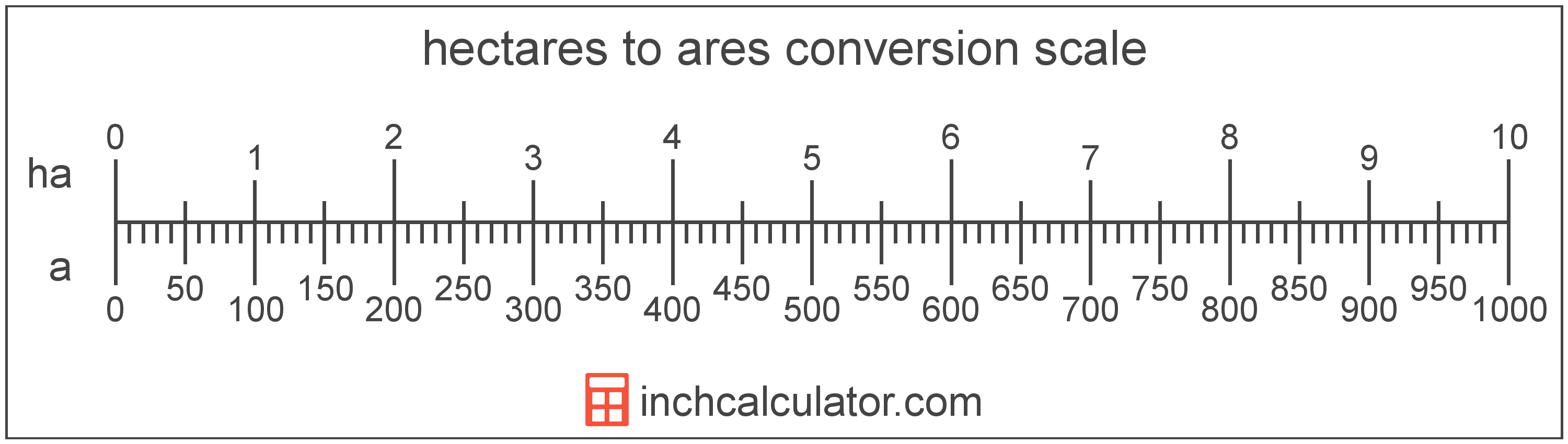# Hectares to Ares Conversion

Enter the area in hectares below to get the value converted to ares.

Results in Ares:1 ha = 100 a
Do you want to convert ares to hectares?

## How to Convert Hectares to Ares

To convert a hectare measurement to an are measurement, multiply the area by the conversion ratio.

Since one hectare is equal to 100 ares, you can use this simple formula to convert:

ares = hectares × 100

The area in ares is equal to the hectares multiplied by 100.

For example, here's how to convert 5 hectares to ares using the formula above.
5 ha = (5 × 100) = 500 a### How Many Ares are in a Hectare?

There are 100 ares in a hectare, which is why we use this value in the formula above.

1 ha = 100 a

## Hectares

One hectare is equal to 10,000 square meters, or the area of a square with 100 meter sides.

The hectare is an SI accepted unit for area for use with the metric system. In the metric system, "hecto" is the prefix for 102. Hectares can be abbreviated as ha; for example, 1 hectare can be written as 1 ha.

## Ares

One are is equal to 100 square meters, or the area of a square with 10 meter sides.

The are is a non-SI metric unit for area. Ares can be abbreviated as a; for example, 1 are can be written as 1 a.

## Hectare to Are Conversion Table

Hectare measurements converted to ares
Hectares Ares
1 ha 100 a
2 ha 200 a
3 ha 300 a
4 ha 400 a
5 ha 500 a
6 ha 600 a
7 ha 700 a
8 ha 800 a
9 ha 900 a
10 ha 1,000 a
11 ha 1,100 a
12 ha 1,200 a
13 ha 1,300 a
14 ha 1,400 a
15 ha 1,500 a
16 ha 1,600 a
17 ha 1,700 a
18 ha 1,800 a
19 ha 1,900 a
20 ha 2,000 a
21 ha 2,100 a
22 ha 2,200 a
23 ha 2,300 a
24 ha 2,400 a
25 ha 2,500 a
26 ha 2,600 a
27 ha 2,700 a
28 ha 2,800 a
29 ha 2,900 a
30 ha 3,000 a
31 ha 3,100 a
32 ha 3,200 a
33 ha 3,300 a
34 ha 3,400 a
35 ha 3,500 a
36 ha 3,600 a
37 ha 3,700 a
38 ha 3,800 a
39 ha 3,900 a
40 ha 4,000 a

## References

1. Cambridge Dictionary, hectare, https://dictionary.cambridge.org/us/dictionary/english/hectare